Windows

# Electric or Magnetic Field Plot

If the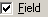box is selected, the energy level plot window will plot the energy levels as a function of electric or magnetic field, rather than as a function of quantum number. Additional controls become visible at the bottom of the control panel to control this plot. Most of the other controls operate the same in both modes, so see the energy level plot window documentation for details.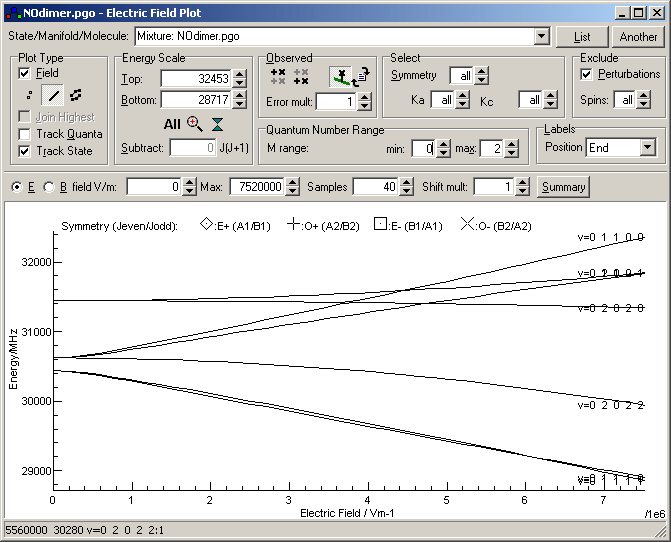## Field Range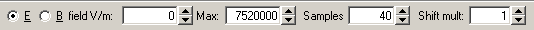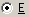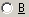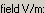Select the field to scan. (If B is selected, the units change to Tesla = 104 Gauss)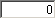Minimum value of field for plot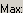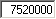Maximum value of field for plot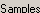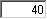Number of fields to calculate the energy levels at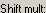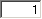Scale field shifts by this factor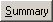Write tuning coefficients to the Log window

## M Range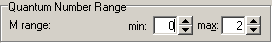The quantum number range selects the range of the projection of the total angular momentum along the field direction, M, that is calculated and plotted.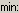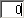Minimum M to include in plot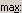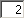Maximum M to include in plot